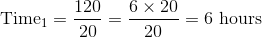## Example Questions

### Example Question #2 : Operations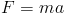in physics where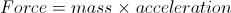.

If the mass is increased by 3 three times the original and the acceleration is increased by 7 times the original, how many times greater is the new force than the original force?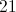Explanation:

We simply multiply the new mass by the new acceleration to obtain a new force that is 21 times greater than the original.

### Example Question #3 : Operations

An office wants to buy 22 computers at $900 each. The budget is$20,000 and the tax on computers is 9%. How many computers can the office afford?Explanation:

The office can only afford 20 computers.

1.09 * 900 = \$981 is the actual price of each computer, with tax.

Divide:

20,000/981 = 20.39

Since the office cannot purchase a partial computer, we round down to 20 computers.

### Example Question #4 : Operations

A paint crew can paintwalls inhours. At this rate, how many walls can the paint crew paint inhours?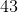Explanation:

First, dividebyto determine how many walls the paint crew can paint inhour.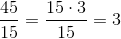divided byis equal to.

Then, multiply this number byhours.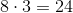### Example Question #2 : New Sat Math Calculator

Convert three yards to inches.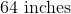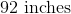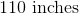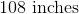Explanation:

To solve this problem, we need to know the conversions between yards to feet, and feet to inches.  Write their correct conversions.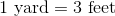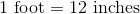Convert three yards to feet.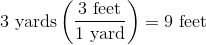Convert nine feet to inches.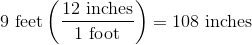### Example Question #1 : How To Multiply Integers

Calculatein the following series: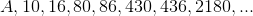Explanation:

Each element is obtained from the previous one by alternating the following operations: multiplying by 5 and adding 6.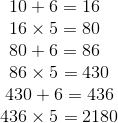If we letbe the first element, then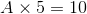Solve for: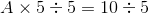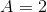### Example Question #2 : How To Multiply Integers

Janet can pick between 10 and 20 tomatoes in an hour. What is a possible amount of time for Janet to pick 120 tomatoes?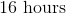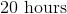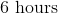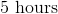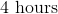Explanation:

Since Janet can pick between 10 and 20 tomatoes in an hour, there is a range for which she can pick 120 tomatoes. Therefore, to find the possible amount of time it will take Janet to pick 120 tomatoes set up two fractions.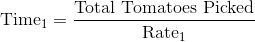The first fraction will use the rate of 10 tomatoes an hour.

Therefore, identifying the variables are as follows.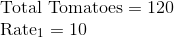Substituting these into the fraction results in a possible time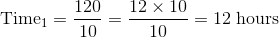The second fraction will use the rate of 20 tomatoes an hour.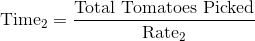Therefore, identifying the variables are as follows.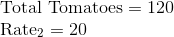Substituting these into the fraction results in a possible time